# Catch up on the latest AI articles# Unsupervised Continuous Learning!

3 main points
✔️ Research on unsupervised continuous learning
✔️ Proposed LUMP to prevent catastrophic forgetting
✔️ Demonstrating the superiority of unsupervised continuous learning over supervised continuous learning

Representational Continuity for Unsupervised Continual Learning
written by Divyam MadaanJaehong YoonYuanchun LiYunxin LiuSung Ju Hwang
(Submitted on 13 Oct 2021 (v1), last revised 15 Oct 2021 (this version, v2))

Subjects: Machine Learning (cs.LG); Computer Vision and Pattern Recognition (cs.CV)code：The images used in this article are from the paper, the introductory slides, or were created based on them.

## first of all

Continual Learning, which aims to learn a set of tasks without forgetting previously acquired knowledge, is an area of research that addresses a critical problem in deep learning: catastrophic forgetting. However, despite active research in this area, existing methods are biased towards Supervised Continual Learning (SCL). Therefore, it may not be practical to apply these methods in the real world, where high-quality labels are difficult to obtain.

In the paper presented in this article, we focus on Unsupervised Continual Learning (UCL), which involves learning representations on a set of unlabeled data while avoiding catastrophic forgetting.

As a result, it is shown that the UCL model has superior properties to the SCL model, such as being more robust against catastrophic forgetting and distribution shifts. We also proposed a simple and effective method called Lifelong Unsupervised Mixup (LUMP), which is an application of mixup to UCL. (For these contributions, this paper has been Accepted (Oral) in ICLR2022.)

### On setting up a continuous learning problem

First, $T$ tasks $\textit{T}_{1:T}=(\textit(T)_1,... ,\textit_T)$, we consider a continuous learning setup where the training is performed on a continuous set of data consisting of $T$ tasks.

For supervised continuous learning (SCL), the task descriptor $\tau \in \{1,... ,T\}$, each task consists of a dataset $D_{tau}=\{(x_{i,\tau},y_{i,\tau})^{n_{\tau}}_{i=1}$ with $n_{\tau}$ examples.

Each input pair is $(X_{i,\tau},Y_{i,\tau})\in X_{\tau}×Y_{\tau}$, where $X_{\tau},Y_{\tau})$ is the unknown data distribution. Here, the feature representation network that transforms the input to embedding is represented as $f_{\Theta}:X_{\tau}→R^D$ (parameter $\Theta=\{w_l\}^{l=L}_{l=1}$, $R^D$ is the $D$-dimensional embedding space and $L$ is the number of layers). Also, the classifier is $h_{\psi}:R^D→Y_{\tau}$.

The cross-entropy loss of the SCL is then expressed by the following equation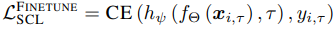On the other hand, since the paper focuses on unsupervised continuous learning (UCL), each task consists of $U_{\tau}=\{(x_{i,\tau})^{n_{\tau}}_{i=1}$. The goal then is to learn a feature representation $f_{\Theta}:X_{\tau}→R^D$ for a set of tasks while preserving knowledge from previous tasks.

Learning Protocols and Assessment Indicators

In the traditional continuous learning strategy, a network representation $f_{\Theta}:X_{\tau}→Y_{\tau}$ is learned in a series of tasks. On the other hand, in the unsupervised continuous learning setting, the goal is to learn $f_{\Theta}:X_{\tau}→R^D$, so the learning protocol is two-stage.

• Stage 1: A series of tasks $T_{1:T}=(\textit{T},... ,\textit{T}_T)$ and acquire a representation.
• Stage 2: The K-nearest neighbor (KNN) classifier is used to evaluate the quality of the pre-trained representations.

If the test accuracy for task $i$ after learning task $T_{\tau}$ is $a_{\tau, i}$, we can define the following two evaluation metrics for the representation obtained by continuous learning.

• Average accuracy: average test accuracy of all tasks completed before training task $\tau$ $A_{\tau}=\frac{1}{\tau}\sum^{\tau}_{i=1}a_{\tau,i}$
• Average Forgetting: the average performance loss between the best accuracy for each task and the accuracy at the end of training $F=\frac{1}{T-1}\sum^{T-1}_{i=1}max_{\tau \in\{1,... ,T\}}(a_{\tau,i}-a_{T,i})$

## On unsupervised continuous learning

### Sequential representation learning through a series of tasks

In the paper, we use SimSiam and BarlowTwins for unsupervised continuous learning, which achieves state-of-the-art performance on standard representation learning benchmarks.

SimSiam is composed of an encoder network $f_{\Theta}$ consisting of a backbone and a Projection MLP $h(\cdot)$ and a Prediction MLP $h(\cdot)$.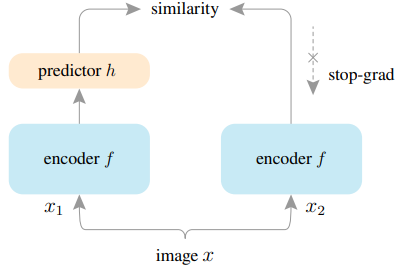SimSiam is trained to minimize the Cosine similarity between the Projector and Predictor output vectors for two different Augmentation processed images $x^1_{i,\tau},x^2_{i,\tau}$.

In this case, for the Predictor output $z^1_{i,\tau}=f_{\Theta}(x^1_{i,\tau})$ and Predictor output $p^2_{i,\tau}=h(f_{\Theta}(x^2_{i,\tau}))$, the objective loss of unsupervised continuous learning is It is expressed as follows.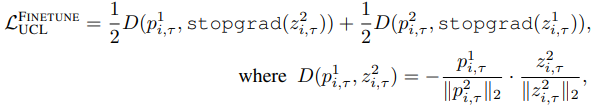BarlowTwins learns to make the cross-correlation matrix computed between the outputs of two identical networks (Encoder+Projectro) close to the identity matrix.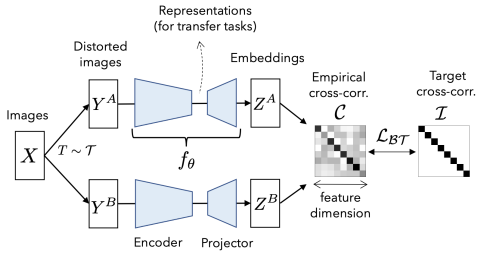Let $C$ be the cross-correlation matrix between the two network outputs, the objective loss of unsupervised continuous learning can be expressed as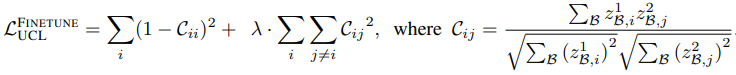Let $\lambda$ denote a positive constant for the trade-off and $i,j$ denote the network output vector dimension.

For both SimSiam and BarlowTwins, it can be applied with little or no change to your existing continuous learning strategy.

### On existing supervised continuous learning methods

In addition to representation learning based on SimSiam and BarlowTwins, we also experiment with examples of extending existing methods for supervised continuous learning to an unsupervised setting. Specifically, we will experiment with the regularization-based method Synaptic Intelligence (SI) and the architecture-based method Progressive Neural Networks (PNN) extended to UCL (i.e., a method that changes the architecture of the model as the learning progresses). Introduction.

We also introduce the following losses as an extension of Dark Experience Replay (DER ) to UCL as a rehearsal-based method (a method to reuse past data in some form).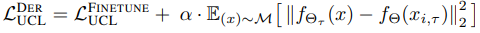where $M$ is the replay buffer.

Also, since the performance of the rehearsal-based method varies significantly depending on the choice of $\alpha$, the paper introduces a Lifelong Unsupervised Mixup to address this issue.

### LUMP (Lifelong Unsupervised Mixup)

In a standard mixup, two random samples $(x_i,y_i),(x_j,y_j)$ are added together with a weight $\lambda$ to create a new sample. In this case, the loss function is expressed by the following equation.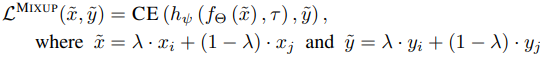Lifelong Unsupervised Mixup (LUMP) uses examples stored in the replay buffer from previous tasks to train the mixup.

That is, for the example $(x_{i,\tau} \in U_{\tau})$ of the current task and the example sampled from the replay buffer, we create an interpolating instance $\tilde{x}{i,\tau}$ expressed as follows.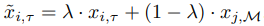where $x_{j, M}~M$ denotes examples sampled from the replay buffer $M$. The $\tilde{x}{i,\tau}$ obtained in this way is used as a sample to learn to minimize $L^{FINETUNE}_{UCL}$.

## experimental setup

### baseline

The experiments compare various supervised/unsupervised continuous learning methods.

SCL (Supervised Continuous Learning)

• FINETUNE: Learning a series of tasks without regularization and episodic memory.
• MULTITASK: train models on complete data.
• Regularization-based methods: SI, AGEM
• Architecture-based method: PNN
• Rehearsal-based methods: GSS, DER

UCL (Unsupervised Continuous Learning)

In unsupervised continuous learning, we use SimSiam and BarlowTwins to learn representations. At this time, we conduct experiments on multiple variants as follows.

• FINETUNE: Same as supervised case
• MULTITASK: Same as supervised case
• Regularization-based method: SI
• Architecture-based method: PNN
• Rehearsal-based methods: an extension for unsupervised DER

data-set

The dataset used in the experiment is as follows

• Split CIFAR-10
• Split CIFAR-100
• Split Tiny-ImageNet

Learning and assessment settings

For the SCL training time, we follow the hyperparameter settings of previous work; the UCL uses an adjusted version of this and is evaluated by the KNN classifier on three independent runs.

The UCL method is trained over 200 epochs. In addition, the hyperparameter settings for each dataset are as follows.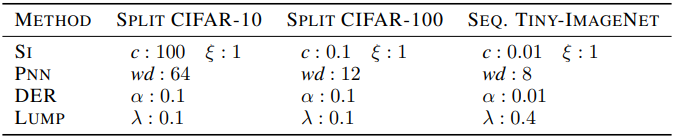## experimental results

The results of the experiment are shown in the table below.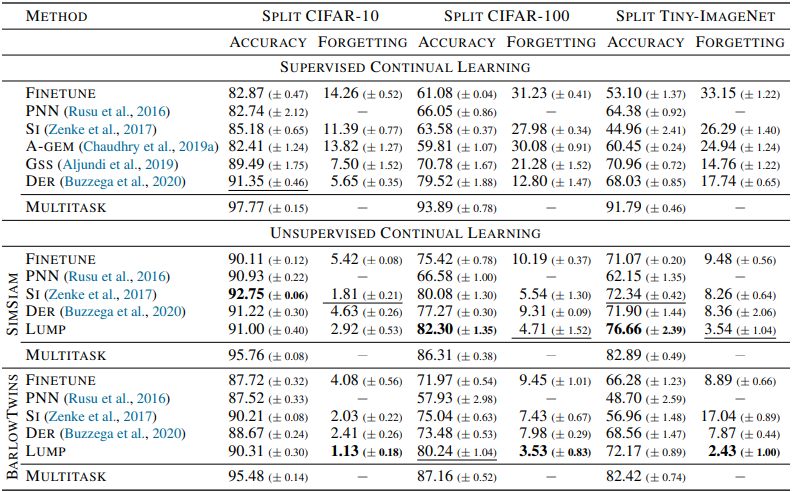In general, unsupervised continuous learning (UCL) showed higher accuracy and lower forgetting rate than the results of supervised continuous learning (SCL).

Interestingly, even the UCL-FINETUNE setting without additional methods such as regularization performed significantly better than supervised continuation learning. The LUMP proposed in the paper also performed significantly better than the other baselines. Furthermore, the results for a small dataset size (few-shot) for each task are as follows.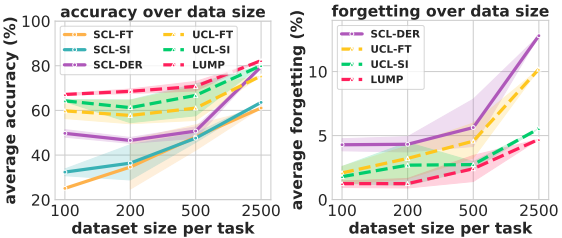In general, the unsupervised method shows significantly better results than the supervised one, especially in terms of accuracy for small data size and forgetfulness for large data size. In addition, the proposed method, LUMP, shows excellent performance in both accuracy and obliviousness. The results of each method on OOD (Out of Distribution) data are also shown below.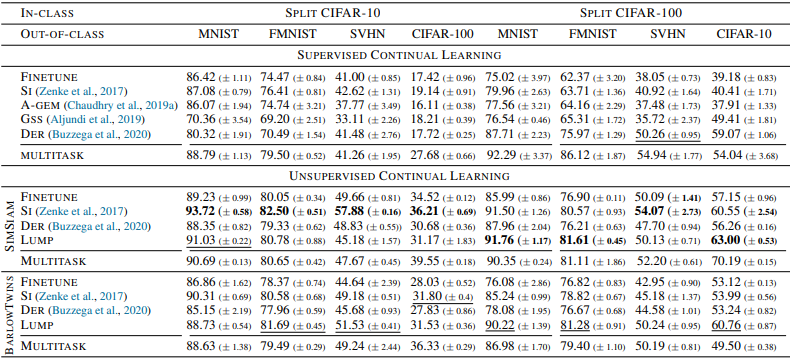In general, the results showed that the representation of unsupervised continuous learning outperformed the supervised setting.

## summary

In this article, we presented our work on unsupervised continuous learning. In this paper, we experimentally demonstrated that representations obtained by unsupervised continuous learning are more robust than those obtained by supervised continuous learning, and proposed a method, LUMP, to suppress catastrophic forgetting. Although there are some issues, such as the limited resolution of the image task used in the experiments, we have shown some useful results on unsupervised continuous learning.

If you have any suggestions for improvement of the content of the article,